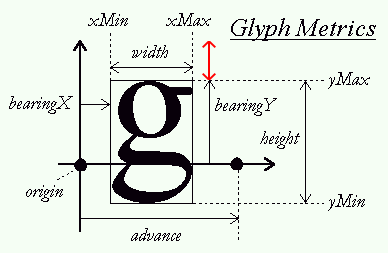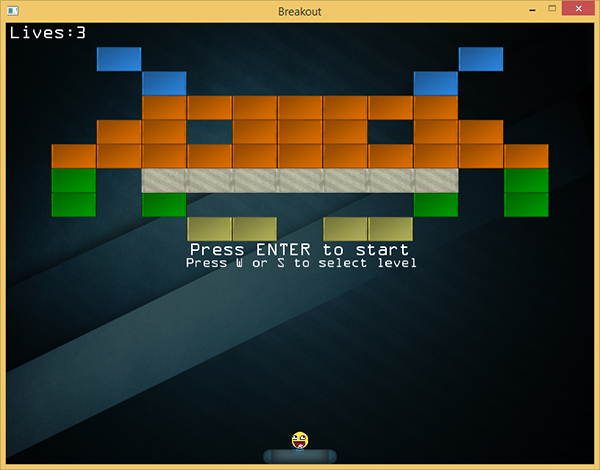# 渲染文本

Note

void TextRenderer::RenderText(std::string text, GLfloat x, GLfloat y, GLfloat scale,glm::vec3 color)
{
[...]
for (c = text.begin(); c != text.end(); c++)
{
GLfloat xpos = x + ch.Bearing.x * scale;
GLfloat ypos = y + (this->Characters['H'].Bearing.y - ch.Bearing.y) * scale;

GLfloat w = ch.Size.x * scale;
GLfloat h = ch.Size.y * scale;
// 为每个字符更新VBO
GLfloat vertices = {
{ xpos,     ypos + h,   0.0, 1.0 },
{ xpos + w, ypos,       1.0, 0.0 },
{ xpos,     ypos,       0.0, 0.0 },

{ xpos,     ypos + h,   0.0, 1.0 },
{ xpos + w, ypos + h,   1.0, 1.0 },
{ xpos + w, ypos,       1.0, 0.0 }
};
[...]
}
}GLfloat ypos = y + (this->Characters['H'].Bearing.y - ch.Bearing.y) * scale;



TextRenderer  *Text;

void Game::Init()
{
[...]
Text = new TextRenderer(this->Width, this->Height);
}



## 玩家生命值

class Game
{
[...]
public:
GLuint Lives;
}


void Game::Update(GLfloat dt)
{
[...]
if (Ball->Position.y >= this->Height) // 球是否接触到底部边界?
{
--this->Lives;
// 玩家是否已失去所有生命值? : 游戏结束
if (this->Lives == 0)
{
this->ResetLevel();
}
this->ResetPlayer();
}
}


void Game::ResetLevel()
{
[...]
this->Lives = 3;
}


void Game::Render()
{
if (this->State == GAME_ACTIVE)
{
[...]
std::stringstream ss; ss << this->Lives;
Text->RenderText("Lives:" + ss.str(), 5.0f, 5.0f, 1.0f);
}
}## 关卡选择

if (this->State == GAME_MENU)
{
if (this->Keys[GLFW_KEY_ENTER])
this->State = GAME_ACTIVE;
if (this->Keys[GLFW_KEY_W])
this->Level = (this->Level + 1) % 4;
if (this->Keys[GLFW_KEY_S])
{
if (this->Level > 0)
--this->Level;
else
this->Level = 3;
}
}


void Game::Render()
{
if (this->State == GAME_ACTIVE || this->State == GAME_MENU)
{
[...] // 游戏状态渲染代码
}
{
Text->RenderText("Press ENTER to start", 250.0f, Height / 2, 1.0f);
Text->RenderText("Press W or S to select level", 245.0f, Height / 2 + 20.0f, 0.75f);
}
}class Game
{
[...]
public:
GLboolean KeysProcessed;
}


void Game::ProcessInput(GLfloat dt)
{
{
if (this->Keys[GLFW_KEY_ENTER] && !this->KeysProcessed[GLFW_KEY_ENTER])
{
this->State = GAME_ACTIVE;
this->KeysProcessed[GLFW_KEY_ENTER] = GL_TRUE;
}
if (this->Keys[GLFW_KEY_W] && !this->KeysProcessed[GLFW_KEY_W])
{
this->Level = (this->Level + 1) % 4;
this->KeysProcessed[GLFW_KEY_W] = GL_TRUE;
}
if (this->Keys[GLFW_KEY_S] && !this->KeysProcessed[GLFW_KEY_S])
{
if (this->Level > 0)
--this->Level;
else
this->Level = 3;
this->KeysProcessed[GLFW_KEY_S] = GL_TRUE;
}
}
[...]
}



void key_callback(GLFWwindow* window, int key, int scancode, int action, int mode)
{
[...]
if (key >= 0 && key < 1024)
{
if (action == GLFW_PRESS)
Breakout.Keys[key] = GL_TRUE;
else if (action == GLFW_RELEASE)
{
Breakout.Keys[key] = GL_FALSE;
Breakout.KeysProcessed[key] = GL_FALSE;
}
}
}



## 获胜

GLboolean GameLevel::IsCompleted()
{
for (GameObject &tile : this->Bricks)
if (!tile.IsSolid && !tile.Destroyed)
return GL_FALSE;
return GL_TRUE;
}



void Game::Update(GLfloat dt)
{
[...]
if (this->State == GAME_ACTIVE && this->Levels[this->Level].IsCompleted())
{
this->ResetLevel();
this->ResetPlayer();
Effects->Chaos = GL_TRUE;
this->State = GAME_WIN;
}
}



void Game::Render()
{
[...]
if (this->State == GAME_WIN)
{
Text->RenderText(
"You WON!!!", 320.0, Height / 2 - 20.0, 1.0, glm::vec3(0.0, 1.0, 0.0)
);
Text->RenderText(
"Press ENTER to retry or ESC to quit", 130.0, Height / 2, 1.0, glm::vec3(1.0, 1.0, 0.0)
);
}
}



void Game::ProcessInput(GLfloat dt)
{
[...]
if (this->State == GAME_WIN)
{
if (this->Keys[GLFW_KEY_ENTER])
{
this->KeysProcessed[GLFW_KEY_ENTER] = GL_TRUE;
Effects->Chaos = GL_FALSE;